# Maxwell's Equations

Maxwell was the first person to calculate the speed of propagation of electromagnetic waves, which was the same as the speed of light and came to the conclusion that EM waves and visible light are similar.

These are the set of partial differential equations that form the foundation of classical electrodynamics, electric circuits and classical optics along with Lorentz force law. These fields highlight modern communication and electrical technologies.

Maxwell’s equations integral form explain how the electric charges and electric currents produce magnetic and electric fields. The equations describe how the electric field can create a magnetic field and vice versa.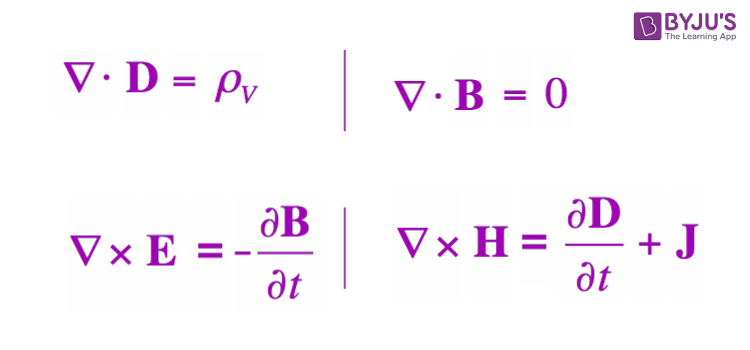### Maxwell First Equation

Maxwell’s first equation is based on the Gauss law of electrostatic, which states that “when a closed surface integral of electric flux density is always equal to charge enclosed over that surface”

Mathematically Gauss law can be expressed as,

Over a closed surface, the product of the electric flux density vector and surface integral is equal to the charge enclosed.

$$\begin{array}{l}∯\vec{D}.d\bar{s}=Q_{enclosed}\, ——(1)\end{array}$$

Any closed system will have multiple surfaces but a single volume. Thus, the above surface integral can be converted into a volume integral by taking the divergence of the same vector. Thus, mathematically it is-

$$\begin{array}{l}∯\bar{D}.d\bar{s}=\iiint \bigtriangledown .\vec{D}d\vec{v}\, —-(2)\end{array}$$

Thus, combining (1) and (2), we get-

$$\begin{array}{l}\iiint \bigtriangledown .\vec{D}d\vec{v}=Q_{enclosed}\, —–(3)\end{array}$$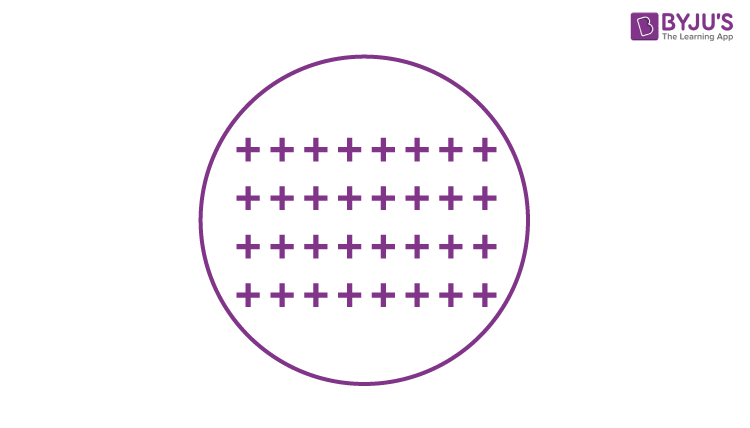Charges in a closed surface will be distributed over its volume. Thus, the volume charge density can be defined as –

$$\begin{array}{l}\rho v=\frac{dQ}{dv}\end{array}$$
measured using C/m3

On rearranging, we get-

$$\begin{array}{l}dQ=\rho vdv\end{array}$$

On integrating the above equation, we get-

$$\begin{array}{l}Q=\iiint \rho vdv\, ——-(4)\end{array}$$

The charge enclosed within a closed surface is given by volume charge density over that volume.

Substituting (4) in (3) we get-

$$\begin{array}{l}\iiint\bigtriangledown .D dv =\iiint \rho vdv\end{array}$$

Canceling the volume integral on both the sides, we arrive at Maxwell’s First Equation-

 $$\begin{array}{l}\Rightarrow \bigtriangledown .D dv = \rho v\end{array}$$

### Maxwell Second Equation

Maxwell second equation is based on Gauss law on magnetostatics.

Gauss law on magnetostatics states that “closed surface integral of magnetic flux density is always equal to total scalar magnetic flux enclosed within that surface of any shape or size lying in any medium.”

Mathematically it is expressed as –

$$\begin{array}{l}∯\vec{B}.ds=\phi_{enclosed}\, —–(1)\end{array}$$
 Scalar Electric Flux (𝜓) Scalar Magnetic Flux (𝜙) They are the imaginary lines of force radiating in an outward direction They are the circular magnetic field generated around a current-carrying conductor. A charge can be source or sink No source/sink

Hence we can conclude that magnetic flux cannot be enclosed within a closed surface of any shape.

$$\begin{array}{l}∯\vec{B}.ds=0\, ———(2)\end{array}$$

Applying the Gauss divergence theorem to equation (2), we can convert it(surface integral)

into volume integral by taking the divergence of the same vector.

$$\begin{array}{l}⇒∯\vec{B}.ds=\iiint \bigtriangledown .\vec{B}dv\, ——–(3)\end{array}$$

Substituting equation (3) in (2) we get-

$$\begin{array}{l}\iiint \bigtriangledown .\vec{B}dv=0\, ——-(4)\end{array}$$

Here to satisfy the above equation either

$$\begin{array}{l}\iiint dv=0\end{array}$$
or
$$\begin{array}{l}\bigtriangledown .\vec{B}=0\end{array}$$
The volume of any body or object can never be zero.

Thus, we arrive at Maxwell’s second equation.

 $$\begin{array}{l}\bigtriangledown .\vec{B}=0\end{array}$$

Where,

$$\begin{array}{l}\vec{B}=\mu \bar{H}\end{array}$$
is the flux density.

$$\begin{array}{l}\Rightarrow \bigtriangledown . \vec{H}=0\end{array}$$
[solonoidal vector is obtained when the divergence of a vector is zero.  Irrotational vector is obtained when the cross product is zero]

### Maxwell Third Equation

Statement: Time-varying magnetic field will always produce an electric field.

Maxwell’s 3rd equation is derived from Faraday’s laws of Electromagnetic Induction. It states that “Whenever there are n-turns of conducting coil in a closed path placed in a time-varying magnetic field, an alternating electromotive force gets induced in each coil.” Lenz’s law gives this. Which states, ” An induced electromotive force always opposes the time-varying magnetic flux.”

When two coils with N number of turns, A primary coil and a Secondary coil. The primary coil is connected to an alternating current source, and the secondary coil is connected in a closed loop and is placed at a small distance from the primary coil. When an AC current passes through the primary coil, an alternating electromotive force gets induced in the secondary coil. See the figure below.

Mathematically it is expressed as –

Alternating emf,

$$\begin{array}{l}emf_{alt}=-N\frac{d\phi }{dt}\, ——–(1)\end{array}$$

Where,

N is the number of turns in a coil.

𝜙 is the scalar magnetic flux.

The negative sign indicates that the induced emf always opposes the time-varying magnetic flux.

Let N=1,

$$\begin{array}{l}\Rightarrow emf_{alt}=-\frac{d\phi }{dt}\, ——-(2)\end{array}$$

Here, the scalar magnetic flux can be replaced by –

$$\begin{array}{l}\phi =\iint \vec{B}.d\vec{s}\, ——–(3)\end{array}$$

Substitute equation (3) in (2)

$$\begin{array}{l}emf_{alt} =-\frac{d}{dt}\iint \vec{B}.d\vec{s}\end{array}$$

Which is a partial differential equation given by-

$$\begin{array}{l}emf_{alt} =\iint -\frac{\delta \vec{B}}{\delta t}.d\vec{s}\, ———–(4)\end{array}$$

The alternating electromotive force induced in a coil is basically a closed path.

$$\begin{array}{l}\Rightarrow emf_{alt} =\oint \vec{E}.d\vec{l}\, ——-(5)\end{array}$$

Substituting equation (5) in (4) we get-

$$\begin{array}{l}\Rightarrow \oint \vec{E}d\vec{l}=\iint -\frac{\delta \vec{B}}{\delta t}.d\vec{s}\, —–(6)\end{array}$$

The closed line integral can be converted into surface integral using Stoke’s theorem. Which states that the “Closed line integral of any vector field is always equal to the surface integral of the curl of the same vector field”

$$\begin{array}{l}\Rightarrow \oint \vec{E}d\vec{l}=\iint \left ( \bigtriangledown \times \vec{E} \right ).d\vec{s}\, ———(7)\end{array}$$

Substituting equation (7) in (6) we get-

$$\begin{array}{l}\Rightarrow \iint \left ( \bigtriangledown \times \vec{E} \right )d\vec{s}=\iint -\frac{\delta \vec{B}}{\delta t}.d\vec{s} ———(8)\end{array}$$
The surface integral can be canceled on both sides. Thus, we arrive at Maxwell’s third equation

 $$\begin{array}{l}\bigtriangledown \times \vec{E}= -\frac{\delta \vec{B}}{\delta t}\end{array}$$

Hence,  we can conclude that the time-varying magnetic field will always produce an electric field.

Extended Maxwell’s third equation of Maxwell’s third equation for the static magnetic field

Which states that the Static electric field vector is an irrotational vector.

Static field implies the time-varying magnetic field is zero,

$$\begin{array}{l}\Rightarrow -\frac{\delta \vec{B}}{\delta t}=0\end{array}$$
$$\begin{array}{l}\Rightarrow \bigtriangledown \times \vec{E}=0\end{array}$$

Hence it is an irrotational vector.

### Maxwell’s Fourth Equation

It is based on Ampere’s circuit law. To understand Maxwell’s fourth equation, it is crucial to understand Ampere’s circuit law,

Consider a wire of a current-carrying conductor with the current I. Since there is an electric field, there has to be a magnetic field vector around it. Ampere’s circuit law states that “The closed line integral of magnetic field vector is always equal to the total amount of scalar electric field enclosed within the path of any shape”, which means the current flowing along the wire(which is a scalar quantity) is equal to the magnetic field vector (which is a vector quantity)

Mathematically it can be written as –

$$\begin{array}{l}\oint \vec{H}.d\vec{l}=I_{enclosed}—–(1)\end{array}$$

Any closed path of any shape or size will occupy one surface area. Thus, L.H.S of equation (1) can be converted into surface integral using Stoke’s theorem, Which states that “Closed line integral of any vector field is always equal to the surface integral of the curl of the same vector field”

$$\begin{array}{l}\oint \vec{H}.d\vec{l}=\iint \left ( \bigtriangledown \times \vec{H} \right ).d\vec{s} —-(2)\end{array}$$

Substituting equation(2) in (1) we get-

$$\begin{array}{l}\iint \left ( \bigtriangledown \times \vec{H} \right ).d\vec{l}=I_{enclosed} ——(3)\end{array}$$

Here,

$$\begin{array}{l}\iint \left ( \bigtriangledown \times \vec{H} \right ).d\vec{l}\,\, is\,\, a \,\,vector \,\,quantity\,\, and\,\,I_{enclosed}\,\,is a scalar quantity.\end{array}$$
$$\begin{array}{l}To \,\,convert\,\, this \,\,scalar \,\,quantity\,\, into\,\, the\,\, vector, \,\,multiplyI_{enclosed}\,\, by\,\, current\,\, density vector \vec{J}. \,\,That\,\, is\,\, defined \,\,by\,\, scalar \,\,current\,\, flowing\,\, per\,\, unit\,\, surface\,\, area.\end{array}$$
$$\begin{array}{l}\vec{J}=\frac{I}{s} \hat{a}N \,\,measured\,\, using\,\, (A/m^2)\end{array}$$

Therefore,

$$\begin{array}{l}\vec{J}=\frac{Difference\;in\;scalar\;electric\;field}{difference\;in\;vector\;surface\;area}\end{array}$$
$$\begin{array}{l}\vec{J}=\frac{dI}{ds}\end{array}$$
$$\begin{array}{l}dI=\vec{J}.d\vec{s}\end{array}$$
$$\begin{array}{l}\Rightarrow I=\iint \vec{J}.d\vec{s} ——-(4)\end{array}$$

Thus, the scalar quantity is converted into vector quantity. Substituting equation(4) into (3) we get-

$$\begin{array}{l}\Rightarrow \iint \left ( \bigtriangledown \times \vec{H} \right ).d\vec{l}=\iint \vec{J}.d\vec{s} ———(5)\end{array}$$

In the above equation, R.H.S and L.H.S both contain surface integral. Hence we can cancel it.

Thus, we arrive at Maxwell’s fourth equation-

 $$\begin{array}{l}\vec{J}=\bigtriangledown \times \vec{H} ——(6)\end{array}$$

We can conclude that the current density vector is a curl of the static magnetic field vector.

On applying the time-varying field(differentiating by time) we get-

$$\begin{array}{l}\bigtriangledown \times\vec{J}=\frac{\delta \rho v}{\delta t} ———(7)\end{array}$$

Apply divergence on both sides of equation(6)-

$$\begin{array}{l}\bigtriangledown .\left ( \bigtriangledown\times \vec{H} \right ) =\bigtriangledown \times\vec{J}\end{array}$$

The divergence of the curl of any vector will always be zero.

$$\begin{array}{l}\bigtriangledown .\left ( \bigtriangledown\times \vec{H} \right ) =0 ——-(8)\end{array}$$

Thus, from equations (7) and(8) we can write that-

$$\begin{array}{l}\frac{\delta \rho v}{\delta t}=0\end{array}$$

Which contradicts the continuity equation for the time-varying fields.

To overcome this drawback we add a general vector to the static field equation(6)

$$\begin{array}{l}\left ( \bigtriangledown \times \vec{H} \right )=\vec{J}+\vec{G}——(9)\end{array}$$

Applying divergence on both sides-

$$\begin{array}{l}\bigtriangledown .\left ( \bigtriangledown \times \vec{H} \right )=\bigtriangledown .\left ( \vec{J}+\vec{G} \right )\end{array}$$

The divergence of the curl of any vector will always be zero.

$$\begin{array}{l}0=\bigtriangledown .\bar{J}+\bigtriangledown .\vec{G}\end{array}$$
$$\begin{array}{l}\bigtriangledown .\vec{G}=-\bigtriangledown .\vec{J} —–(10)\end{array}$$

Substituting equation(6) in (10) we get-

$$\begin{array}{l}\bigtriangledown .\vec{G}=\frac{\delta \rho v}{\delta t} —–(11)\end{array}$$

By Maxwell’s first equation,

$$\begin{array}{l}\rho v=\bigtriangledown .\vec{D}\end{array}$$

Substituting the value of ρv in equation (11) we get-

$$\begin{array}{l}\bigtriangledown .\vec{G}=\frac{\delta \left ( \bigtriangledown .\vec{D} \right )}{\delta t} —–(12)\end{array}$$

Here,

$$\begin{array}{l}\frac{\delta }{\delta t}\,\,is \,\,time\,\, varient\,\, and\end{array}$$
$$\begin{array}{l}\bigtriangledown .\vec{D}\end{array}$$
is space variant and both are independent to each other. Thus, on rearranging equation (12) we get-

$$\begin{array}{l}\bigtriangledown .\vec{G}= \bigtriangledown .\frac{\delta \left (\vec{D} \right )}{\delta t}\end{array}$$

Thus cancelling the like terms we get-

$$\begin{array}{l}\vec{G}= \frac{\delta \vec{D}}{\delta t}=\vec{J}_{D} —–(13)\end{array}$$

Substituting them in

$$\begin{array}{l}\left ( \bigtriangledown \times \vec{H} \right )=\vec{J}+\vec{G}\end{array}$$

This is an insulating current flowing in the dielectric medium between two conductors.

Hence maxwell’s fourth equation will be

$$\begin{array}{l}\Rightarrow \left ( \bigtriangledown \times \vec{H} \right )=\vec{J}+\vec{J}_{D}\end{array}$$

Or

 $$\begin{array}{l}\Rightarrow \left ( \bigtriangledown \times \vec{H} \right )=\vec{J}+\frac{\delta\vec{D} }{\delta t}\end{array}$$

Where,

$$\begin{array}{l}\vec{J}_{D}\,\,is \,\,Displacement \,\,current \,\,density. \end{array}$$

We know that magnetic flux is equal to the product of the electric flux and permittivity.

$$\begin{array}{l}\vec{D}= \varepsilon \vec{E} —–(14)\end{array}$$

Substituting equation (14) in (13) we get-

$$\begin{array}{l}\frac{\delta \left ( \varepsilon \vec{E} \right )}{\delta t}=\vec{J}_{D}\end{array}$$
$$\begin{array}{l}\Rightarrow \vec{J}_{D}=\varepsilon \frac{\delta \left ( \vec{E} \right )}{\delta t}\end{array}$$
1. ## Gauss Law

Gauss law describes the nature of the electric field around electric charges. The law is expressed in terms of electric charge density and electric charge density.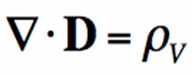The inverted triangle is called the divergence operator.

The equations hold good at any point in space. When the electric charge exists any somewhere, the divergence of D at that particular point is nonzero, else it is zero.

1. ## Gauss’ Magnetism Law

You need to be familiar with Gauss Law for the electric field to understand this equation.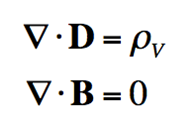You can see that both the equations indicate the divergence of the field. The top equation states that the divergence of the electric flux density D equals the volume of electric charge density.

The second equation states the divergence of the Magnetic Flux Density (B) is null.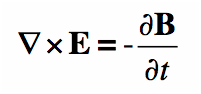Faraday was a scientist whose experiment setup led to Faraday’s Law which is shown in the figure below.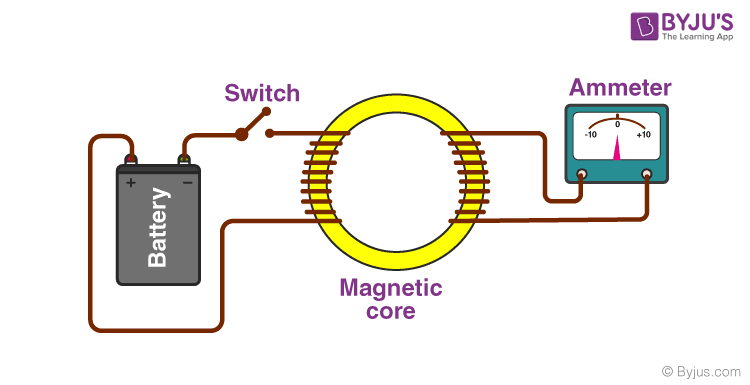The experiment is not very complex. When a battery is disconnected, no electricity flows through the wire. Hence, no magnetic flux is induced in the iron (Magnetic Core). The iron acts like a magnetic field that flows easily in a magnetic material. The purpose of the core is to form a path for the flow of magnetic flux.

## Ampere’s Law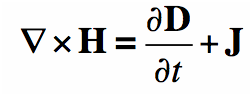The law shows the relationship between the flow of electric current and the magnetic field around it. Suppose the wire carries a current I, the current produces a magnetic field that surrounds the wire.

### Video on Maxwell’s Equations

You will learn about the four Maxwell’s equation with help of animations in the video.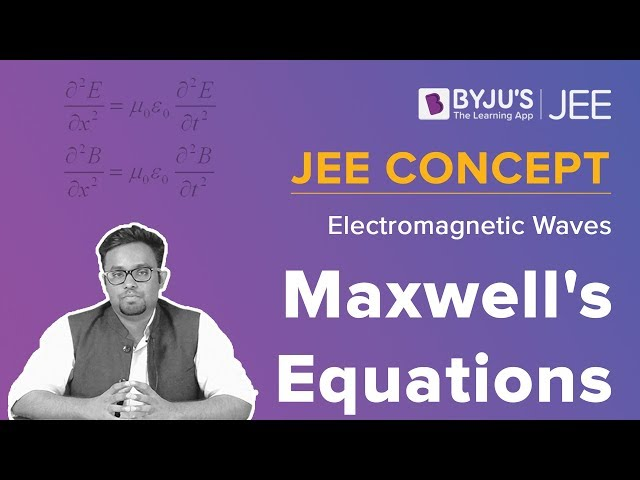## Frequently Asked Questions – FAQs

Q1

### What are electromagnetic waves?

Waves that are created due to the vibrations between a magnetic field and an electric field are known as electromagnetic waves.

Q2

### What does the Maxwell’s equations explain?

It explains how the electric charges and electric currents produce magnetic and electric fields. Maxwell’s equations describe how the electric field can create a magnetic field and vice versa.

Q3

### What does Maxwell third equation state?

Maxwell third equation states that, time-varying magnetic field will always produce an electric field.

Q4

### Give the formula of Gauss’ Magnetism Law.

$$\begin{array}{l}\bigtriangledown . D = \rho _{v}\end{array}$$
$$\begin{array}{l}\bigtriangledown . B = 0\end{array}$$
Q5

### What is meant by scalar electric flux?

Scalar electric flux are the imaginary lines of force radiating in an outward direction.

To know more about problems on Maxwell’s Law along with solved examples, visit BYJU’S.